# SimpleDateFormat 线程不安全的 5 种解决方案！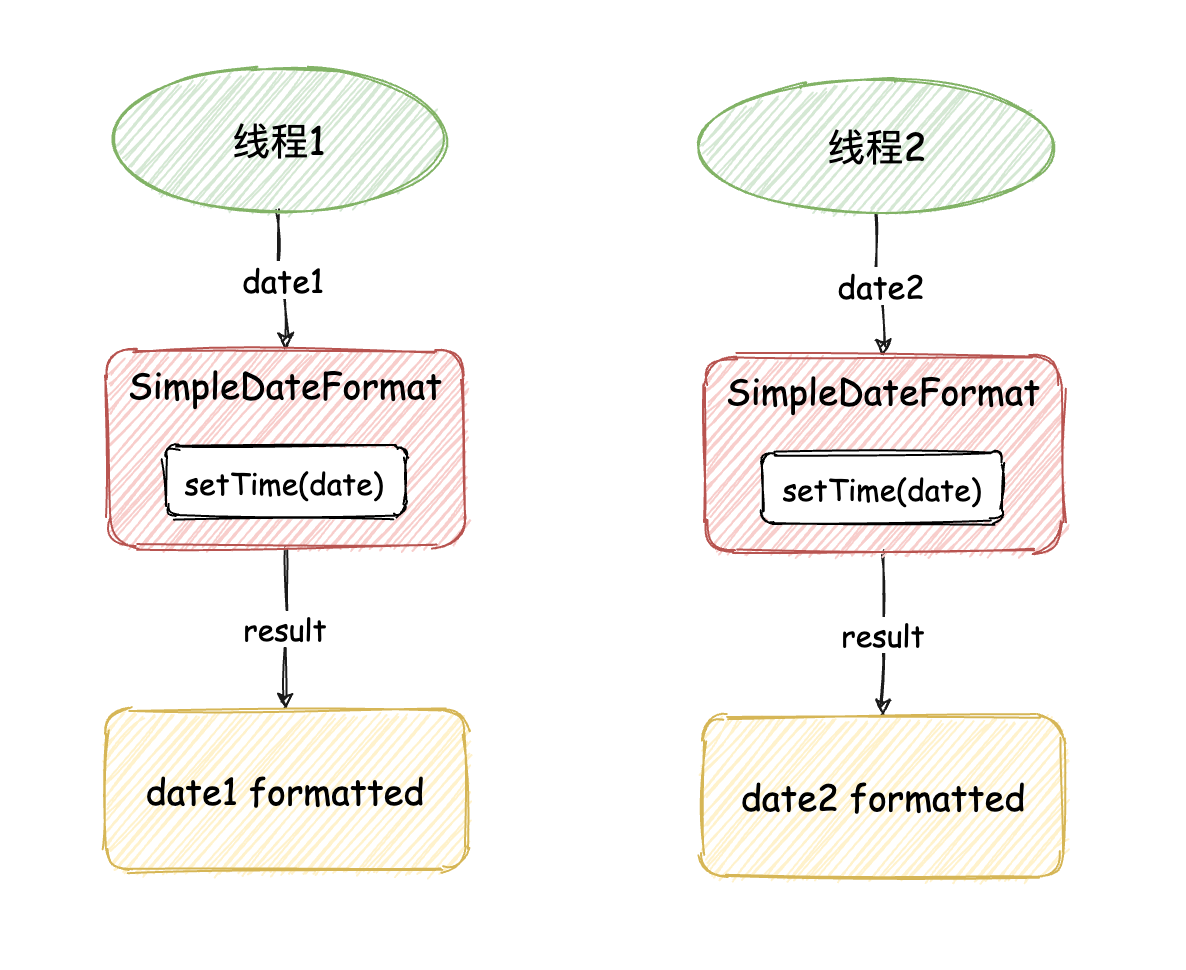## 1.什么是线程不安全？

### 线程不安全的代码

SimpleDateFormat 就是一个典型的线程不安全事例，接下来我们动手来实现一下。首先我们先创建 10 个线程来格式化时间，时间格式化每次传递的待格式化时间都是不同的，所以程序如果正确执行将会打印 10 个不同的值，接下来我们来看具体的代码实现：

import java.text.SimpleDateFormat;import java.util.Date;import java.util.concurrent.ExecutorService;import java.util.concurrent.Executors;public class SimpleDateFormatExample {    // 创建 SimpleDateFormat 对象    private static SimpleDateFormat simpleDateFormat = new SimpleDateFormat("mm:ss");    public static void main(String[] args) {        // 创建线程池        ExecutorService threadPool = Executors.newFixedThreadPool(10);        // 执行 10 次时间格式化        for (int i = 0; i < 10; i++) {            int finalI = i;            // 线程池执行任务            threadPool.execute(new Runnable() {                @Override                public void run() {                    // 创建时间对象                    Date date = new Date(finalI * 1000);                    // 执行时间格式化并打印结果                    System.out.println(simpleDateFormat.format(date));                }            });        }    }}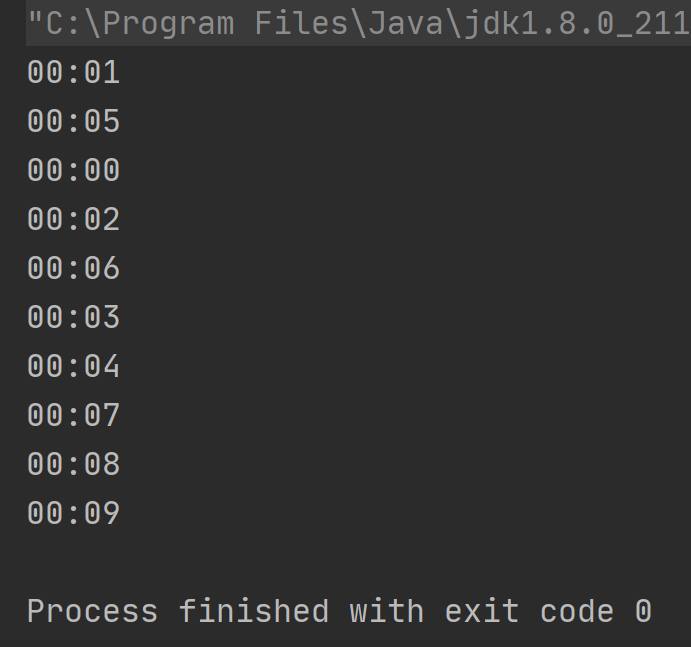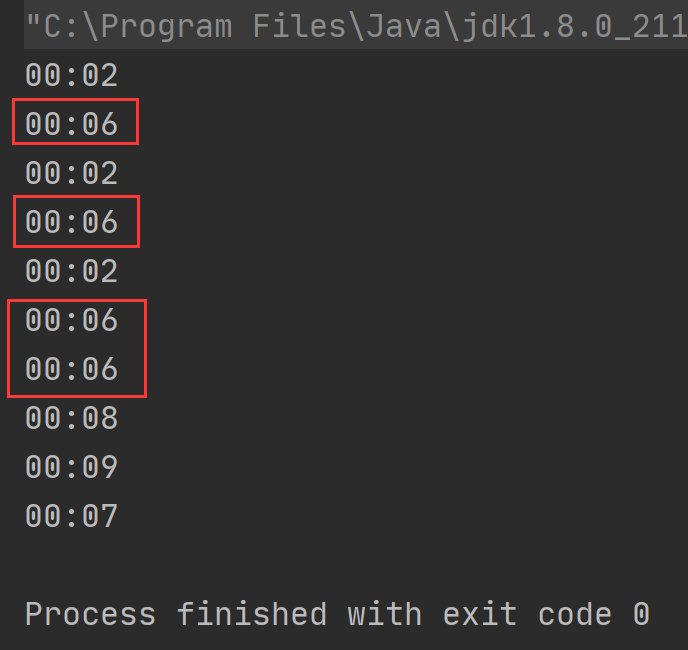## 2.解决方案

SimpleDateFormat 线程不安全的解决方案总共包含以下 5 种：

1. SimpleDateFormat 定义为局部变量；

2. 使用 synchronized 加锁执行；

3. 使用 Lock 加锁执行（和解决方案 2 类似）；

4. 使用 ThreadLocal

5. 使用 JDK 8 中提供的 DateTimeFormat

### ① 将 SimpleDateFormat 变为局部变量

SimpleDateFormat 定义为局部变量时，因为每个线程都是独享 SimpleDateFormat 对象的，相当于将多线程程序变成“单线程”程序了，所以不会有线程不安全的问题，具体实现代码如下：

import java.text.SimpleDateFormat;import java.util.Date;import java.util.concurrent.ExecutorService;import java.util.concurrent.Executors;public class SimpleDateFormatExample {    public static void main(String[] args) {        // 创建线程池        ExecutorService threadPool = Executors.newFixedThreadPool(10);        // 执行 10 次时间格式化        for (int i = 0; i < 10; i++) {            int finalI = i;            // 线程池执行任务            threadPool.execute(new Runnable() {                @Override                public void run() {                    // 创建 SimpleDateFormat 对象                    SimpleDateFormat simpleDateFormat = new SimpleDateFormat("mm:ss");                    // 创建时间对象                    Date date = new Date(finalI * 1000);                    // 执行时间格式化并打印结果                    System.out.println(simpleDateFormat.format(date));                }            });        }        // 任务执行完之后关闭线程池        threadPool.shutdown();    }}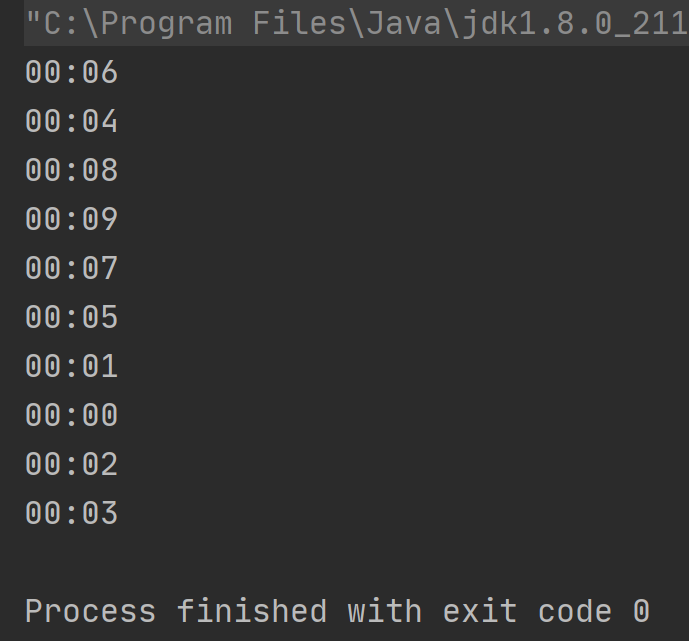### ② 使用 synchronized 加锁

import java.text.SimpleDateFormat;import java.util.Date;import java.util.concurrent.ExecutorService;import java.util.concurrent.Executors;public class SimpleDateFormatExample2 {    // 创建 SimpleDateFormat 对象    private static SimpleDateFormat simpleDateFormat = new SimpleDateFormat("mm:ss");    public static void main(String[] args) {        // 创建线程池        ExecutorService threadPool = Executors.newFixedThreadPool(10);        // 执行 10 次时间格式化        for (int i = 0; i < 10; i++) {            int finalI = i;            // 线程池执行任务            threadPool.execute(new Runnable() {                @Override                public void run() {                    // 创建时间对象                    Date date = new Date(finalI * 1000);                    // 定义格式化的结果                    String result = null;                    synchronized (simpleDateFormat) {                        // 时间格式化                        result = simpleDateFormat.format(date);                    }                    // 打印结果                    System.out.println(result);                }            });        }        // 任务执行完之后关闭线程池        threadPool.shutdown();    }}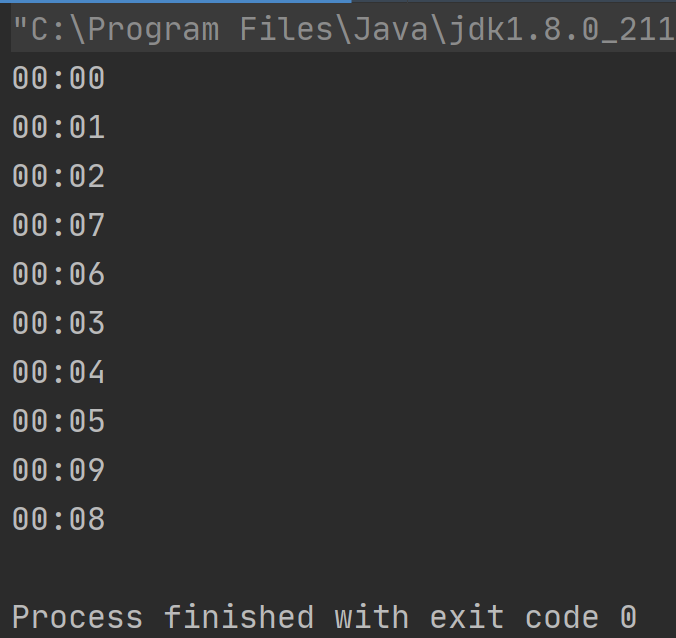### ③ 使用 Lock 加锁

import java.text.SimpleDateFormat;import java.util.Date;import java.util.concurrent.ExecutorService;import java.util.concurrent.Executors;import java.util.concurrent.locks.Lock;import java.util.concurrent.locks.ReentrantLock;/** * Lock 解决线程不安全问题 */public class SimpleDateFormatExample3 {    // 创建 SimpleDateFormat 对象    private static SimpleDateFormat simpleDateFormat = new SimpleDateFormat("mm:ss");    public static void main(String[] args) {        // 创建线程池        ExecutorService threadPool = Executors.newFixedThreadPool(10);        // 创建 Lock 锁        Lock lock = new ReentrantLock();        // 执行 10 次时间格式化        for (int i = 0; i < 10; i++) {            int finalI = i;            // 线程池执行任务            threadPool.execute(new Runnable() {                @Override                public void run() {                    // 创建时间对象                    Date date = new Date(finalI * 1000);                    // 定义格式化的结果                    String result = null;                    // 加锁                    lock.lock();                    try {                        // 时间格式化                        result = simpleDateFormat.format(date);                    } finally {                        // 释放锁                        lock.unlock();                    }                    // 打印结果                    System.out.println(result);                }            });        }        // 任务执行完之后关闭线程池        threadPool.shutdown();    }}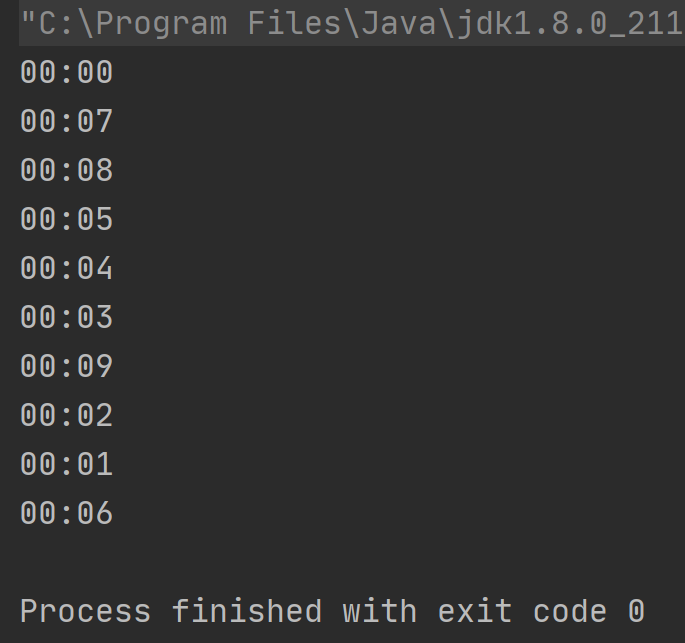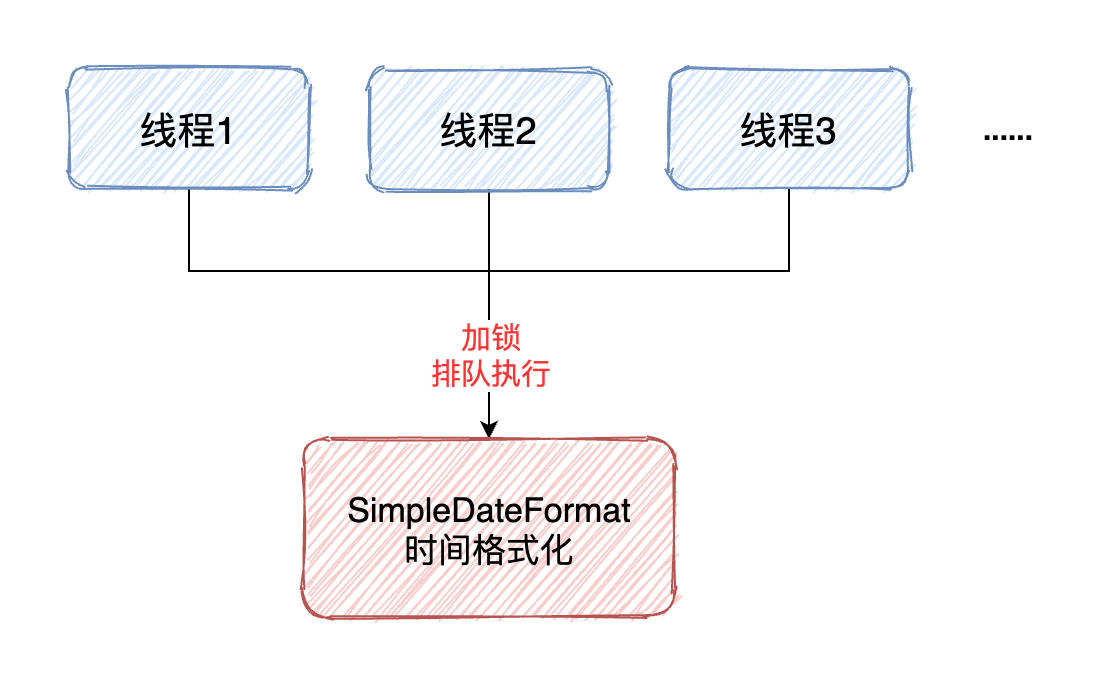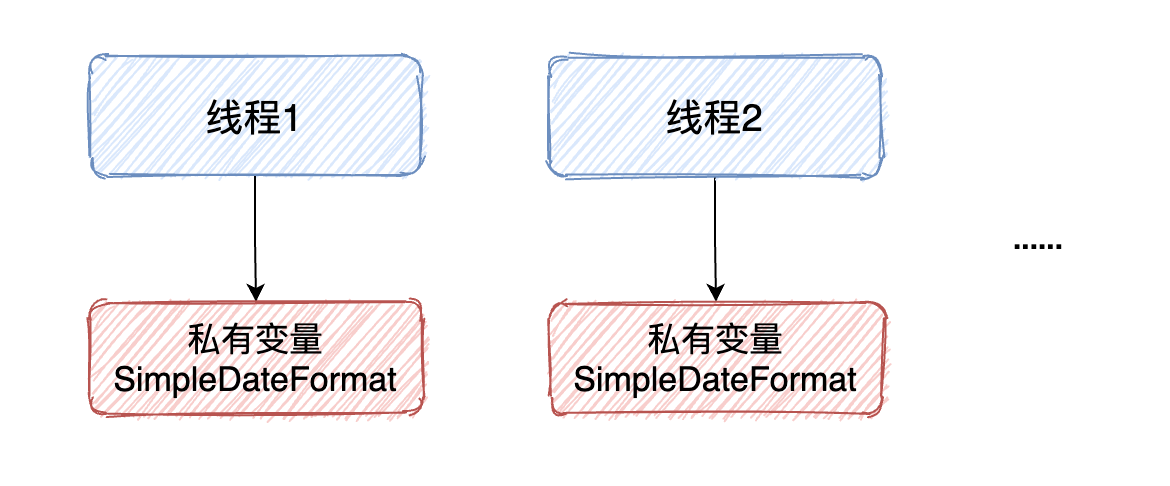import java.text.SimpleDateFormat;import java.util.Date;import java.util.concurrent.ExecutorService;import java.util.concurrent.Executors;/** * ThreadLocal 解决线程不安全问题 */public class SimpleDateFormatExample4 {    // 创建 ThreadLocal 对象，并设置默认值（new SimpleDateFormat）    private static ThreadLocal<SimpleDateFormat> threadLocal =            ThreadLocal.withInitial(() -> new SimpleDateFormat("mm:ss"));    public static void main(String[] args) {        // 创建线程池        ExecutorService threadPool = Executors.newFixedThreadPool(10);        // 执行 10 次时间格式化        for (int i = 0; i < 10; i++) {            int finalI = i;            // 线程池执行任务            threadPool.execute(new Runnable() {                @Override                public void run() {                    // 创建时间对象                    Date date = new Date(finalI * 1000);                    // 格式化时间                    String result = threadLocal.get().format(date);                    // 打印结果                    System.out.println(result);                }            });        }        // 任务执行完之后关闭线程池        threadPool.shutdown();    }}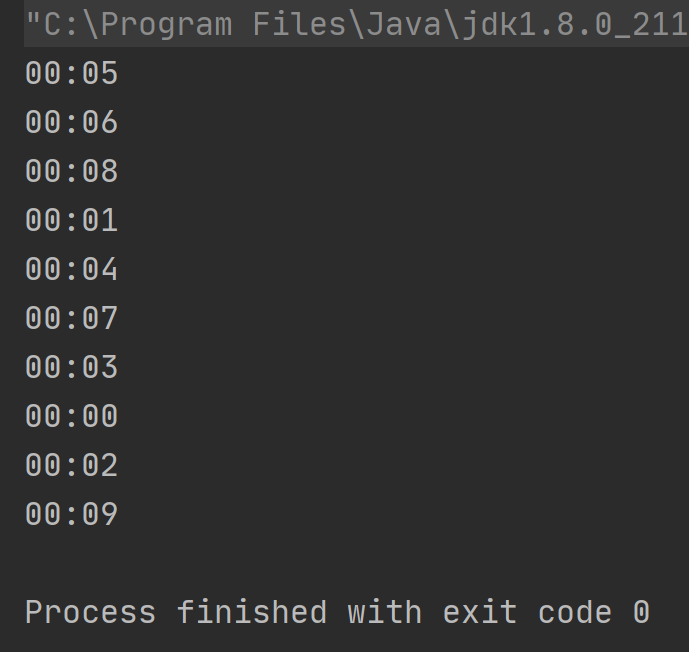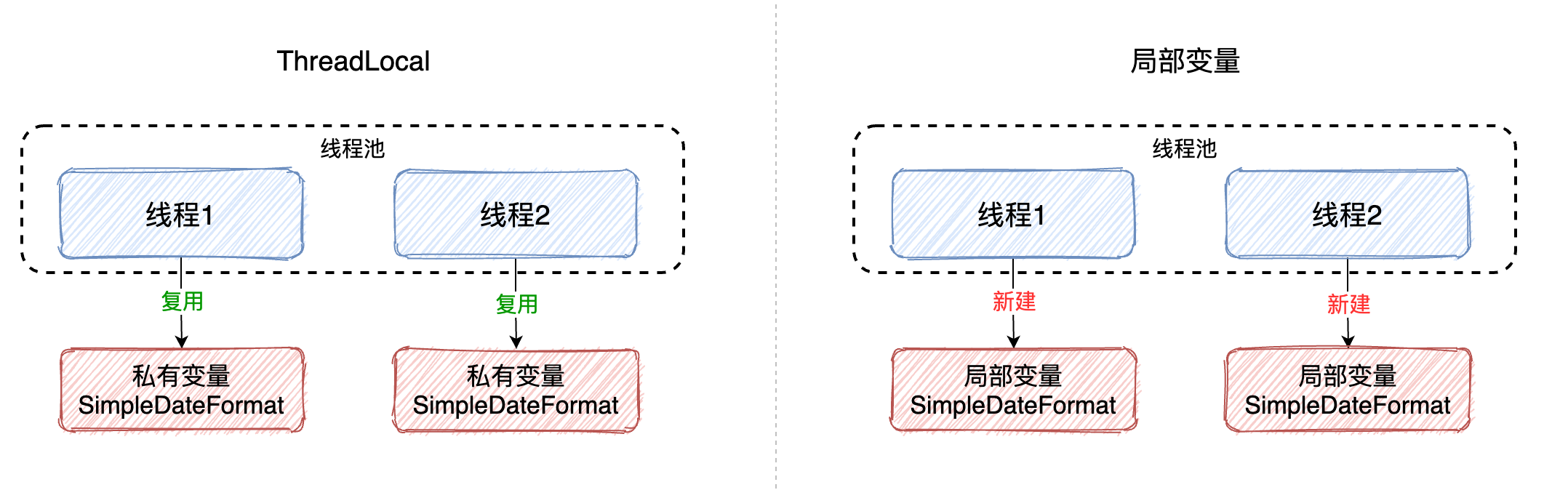### ⑤ 使用 DateTimeFormatter

import java.time.LocalDateTime;import java.time.ZoneId;import java.time.format.DateTimeFormatter;import java.util.Date;import java.util.concurrent.ExecutorService;import java.util.concurrent.Executors;/** * DateTimeFormatter 解决线程不安全问题 */public class SimpleDateFormatExample5 {    // 创建 DateTimeFormatter 对象    private static DateTimeFormatter dateTimeFormatter = DateTimeFormatter.ofPattern("mm:ss");    public static void main(String[] args) {        // 创建线程池        ExecutorService threadPool = Executors.newFixedThreadPool(10);        // 执行 10 次时间格式化        for (int i = 0; i < 10; i++) {            int finalI = i;            // 线程池执行任务            threadPool.execute(new Runnable() {                @Override                public void run() {                    // 创建时间对象                    Date date = new Date(finalI * 1000);                    // 将 Date 转换成 JDK 8 中的时间类型 LocalDateTime                    LocalDateTime localDateTime =                            LocalDateTime.ofInstant(date.toInstant(), ZoneId.systemDefault());                    // 时间格式化                    String result = dateTimeFormatter.format(localDateTime);                    // 打印结果                    System.out.println(result);                }            });        }        // 任务执行完之后关闭线程池        threadPool.shutdown();    }}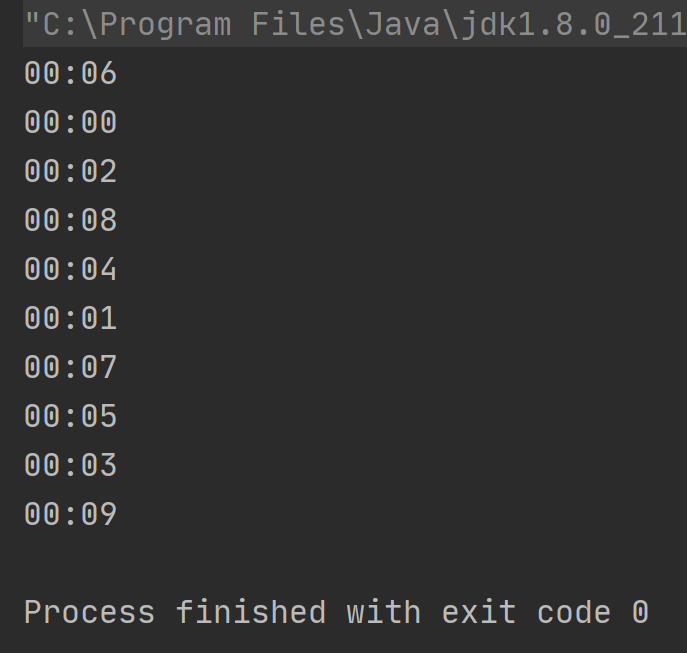## 3.线程不安全原因分析

private StringBuffer format(Date date, StringBuffer toAppendTo,                                FieldDelegate delegate) {    // 注意此行代码    calendar.setTime(date);    boolean useDateFormatSymbols = useDateFormatSymbols();    for (int i = 0; i < compiledPattern.length; ) {        int tag = compiledPattern[i] >>> 8;        int count = compiledPattern[i++] & 0xff;        if (count == 255) {            count = compiledPattern[i++] << 16;            count |= compiledPattern[i++];        }        switch (tag) {            case TAG_QUOTE_ASCII_CHAR:                toAppendTo.append((char)count);                break;            case TAG_QUOTE_CHARS:                toAppendTo.append(compiledPattern, i, count);                i += count;                break;            default:                subFormat(tag, count, delegate, toAppendTo, useDateFormatSymbols);                break;        }    }    return toAppendTo;}

1. 线程 1 执行了 calendar.setTime(date) 方法，将用户输入的时间转换成了后面格式化时所需要的时间；

2. 线程 1 暂停执行，线程 2 得到 CPU 时间片开始执行；

3. 线程 2 执行了 calendar.setTime(date) 方法，对时间进行了修改；

4. 线程 2 暂停执行，线程 1 得出 CPU 时间片继续执行，因为线程 1 和线程 2 使用的是同一对象，而时间已经被线程 2 修改了，所以此时当线程 1 继续执行的时候就会出现线程安全的问题了。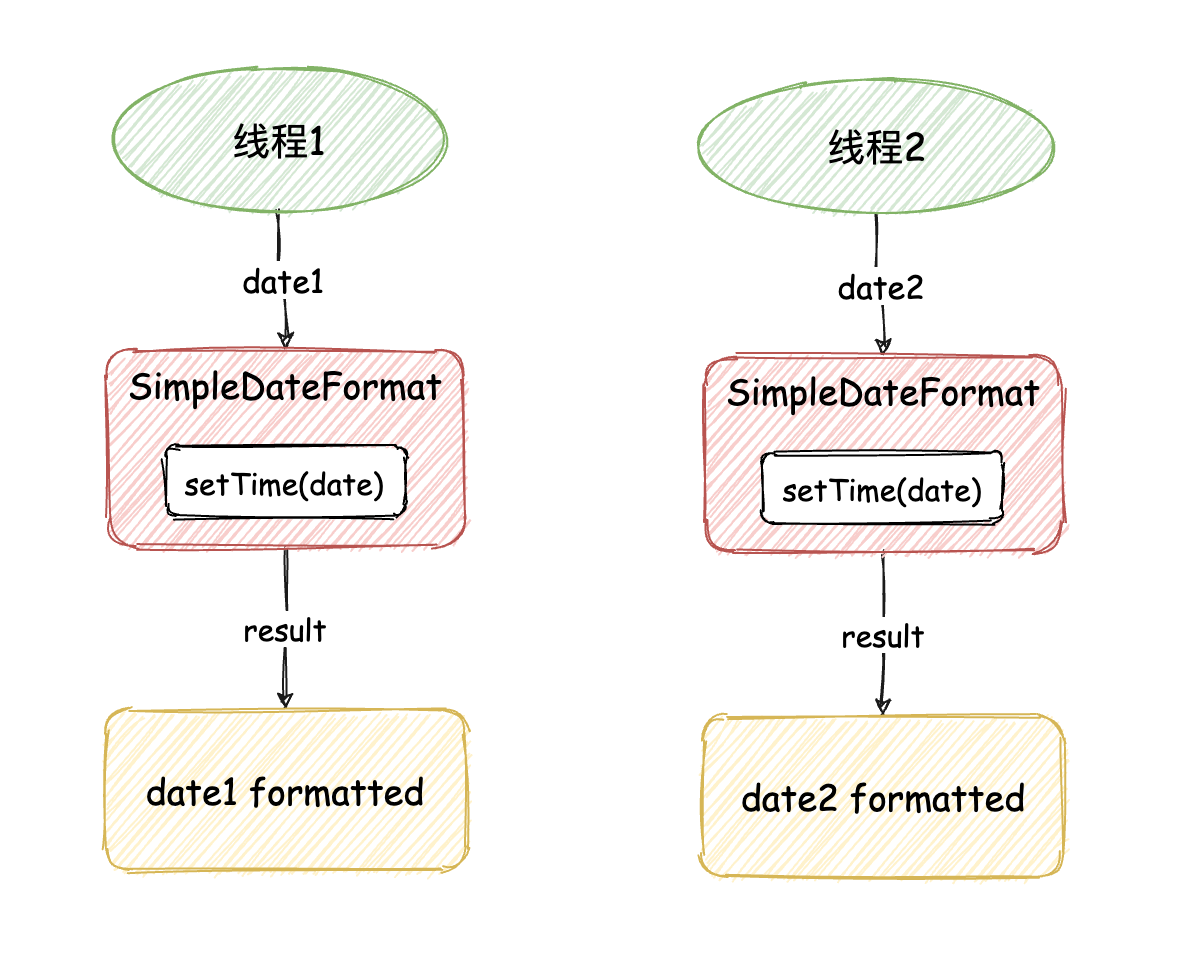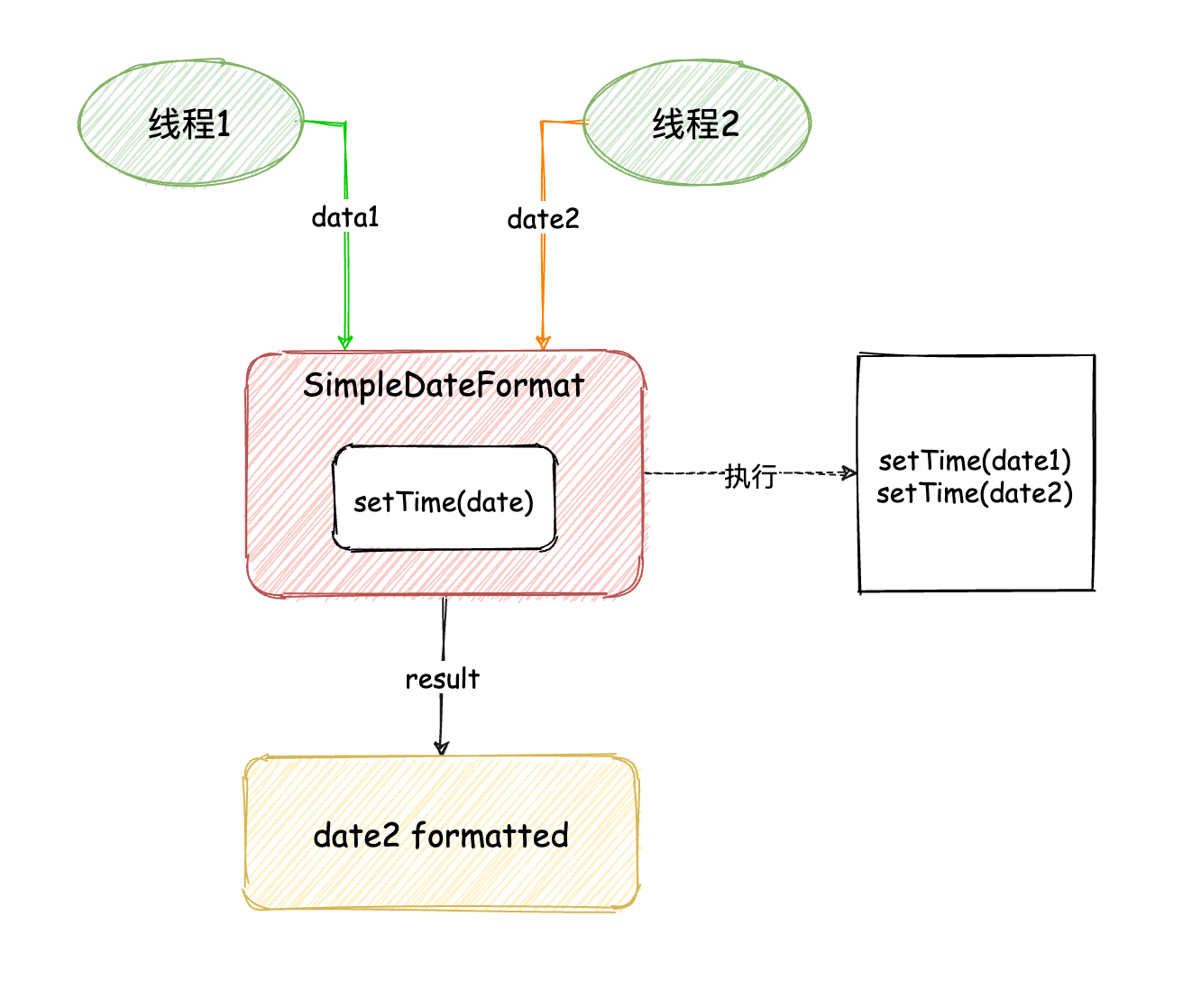## 4.各方案优缺点总结## 评论